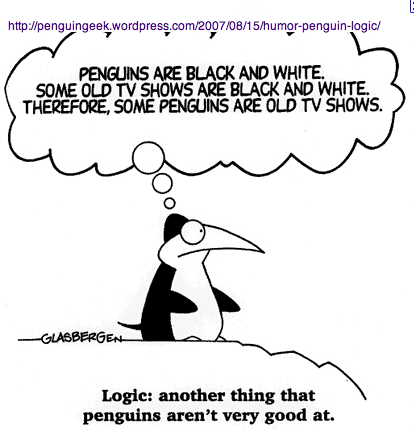Last edited 27jan16

Minal Summary of the Logic Lesson

\begin{document}
\maketitleThis page is the shortest possible summary the lesson on Logic. It does not
substitute for your studying the other two lessons
Commentary on Chapter 2 of D'Angel-West Text
and
Truth Tables, or from doing the filecard questions to test your comprehension of this material.

There are three basic concepts in the lesson:
\begin{itemize}
\item  Proposition and the Propositional Calculus.
\item  Sets and Boolean Algebra of Sets.
\item  Quantifiers and the Predicate Calcuus.
\end{itemize}
and how they are related to each other. In addition, here to may learn or
review what you need to know about the mathematical notation involved.

A sentence is a linguistic phrase with a subject and a predicate. But a
proposition is a sentence that has a truth value, written 1 for "true" and
0 for "false". A sentence is not a proposition if it is not possible to
determine its truth value, either for you or anybody. Russel's example
The king of France is bald is not a proposition, because there is
no king of France at the present time.

The propositional calculus is about putting using propositions and
connectivs to make new propositions. A truth table for a connective
is a function whose output is the truth value based on the truth value(s)
of the input propositions. For example $A \wedge B$ and $\neg A$.

Truth values can also be used in verifying equivalences. For example
\begin{eqnarray*}
\mbox{To show} \neg(A \wedge B) & \sim & \neg A \vee \neg B \\
\mbox{if} A=0 \mbox{then}  \neg(0 \wedge B) & ?  & \neg 0 \vee \neg B   \\
\neg(0) &? & 1 \vee \neg B \\
1  &= & 1 \\
\mbox{if} A=1 \mbox{then}  \neg(1 \wedge B) & ?  & \neg 1 \vee \neg B \\
\neg B &? & \neg 1 \vee \neg B \\
\neg B  &= & \neg B \\
\end{eqnarray*}

A mathematical set differs from just a collection of objects in that there
is a criterion which determines whether something is a an element of the
set or not.  We write this proposition as $x\in A$, which is true if $x$
is an element of $A$ and otherwise it is false, $x \notin A$.

For example, the tautology $A = \{ x | x \in A\}$ is a true proposition
because any object $x \in A$ if and only if the criterion $x \in A$ is true.
However, despite millennia of efforts by philosophers, the totality of
good persons is not a set because it is not possible to determine
that a particular person is good person.

A better example of a non-set is  Russel's Paradox .  Let us define
a set to be ordinary if it is not a member of itself, $A\notin A$.
If it is a member of itself, $A\in A$, we call it extraordinary.
For example, "the set of things that can be described in fewer than
twenty words" is extraordinary.

A logical problem arises with the set of all ordinary sets,
$R=\{ X | X \notin X\}$. Is it ordinary or extraordinary? If $R \notin R$
(it is not ordinary), then
it should be an element of $R$, $R\in R$, which contradicts the assumption.
But if $R \in R$ (it extraordinary) then it
is excluded from $R$, again a contradiction. Even though there is a
perfectly good criterion for being in the collection of all ordinary
sets, after all $R\in R$ is either true or false, it should not be
considered a set.

But have no fear. Russel's paradox does not apply to MA347 because
we shall never consider such comprehensive collections. We mainly
consider sets of numbers which are immune.

An example of this is complement of a set,
written and defined by $\overline A = \{ x | x \notin A \}$.

Two sets are the same provided they have the same membership.
For example
\begin{eqnarray*}
\mbox{To show} \overline{A\cupB}& =& \overline{A} \cap \overline{B} \\
\mbox{First show that   }LHS & \subset& RHS \\
x \in LHS &\Rightarrow& x \notin A \cup B \\
&\Rightarrow& x \notin A  \wedge x \notin B \\
&\Rightarrow& x \in \neg A  \wedge x \in \neg B \\
\mbox{Then show that   }RHS & \subset LHS \\
\end{eqnarray*}
We leave it to you to complete this proof to let you know you have
understood. If not, study the lesson again.

(The third item, predicate calulus, is not summarized here yet.)

\end{document}